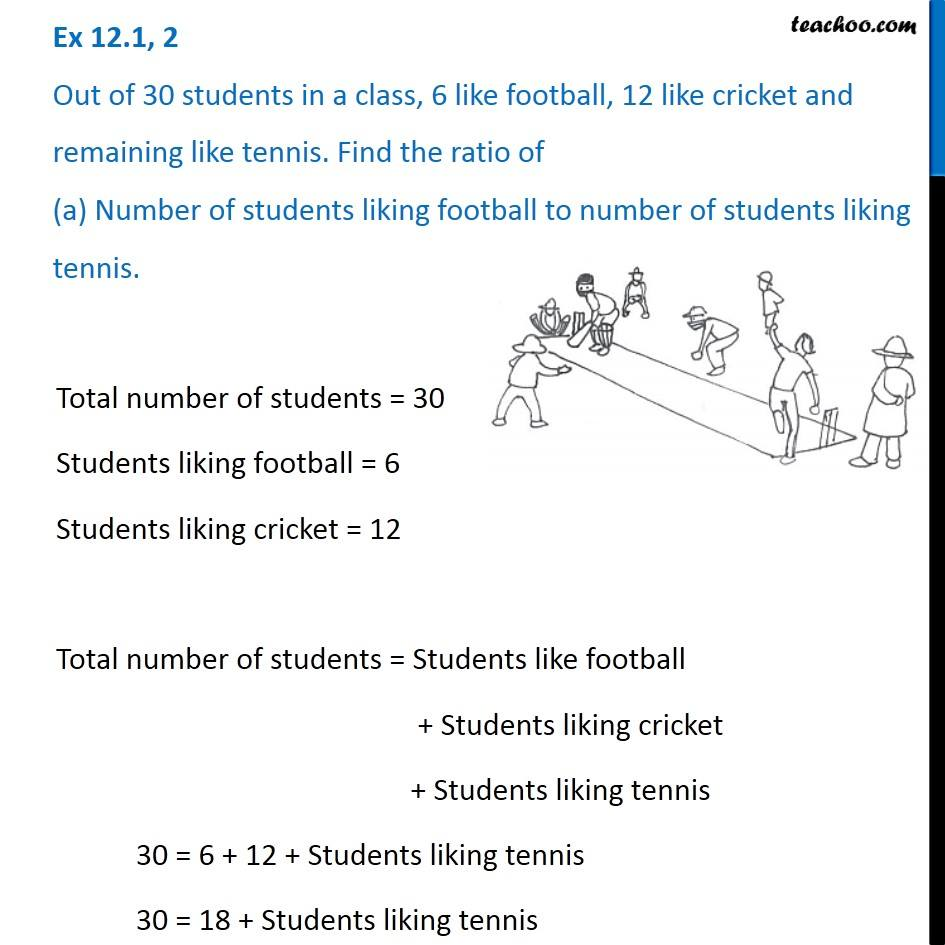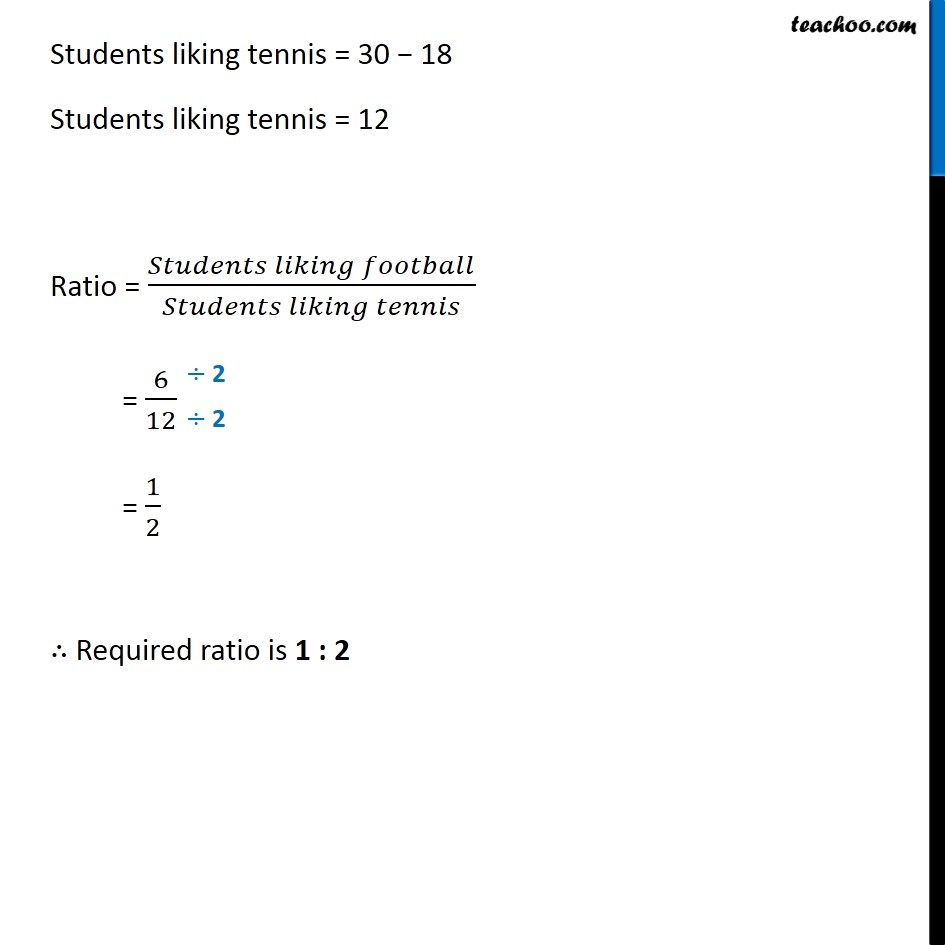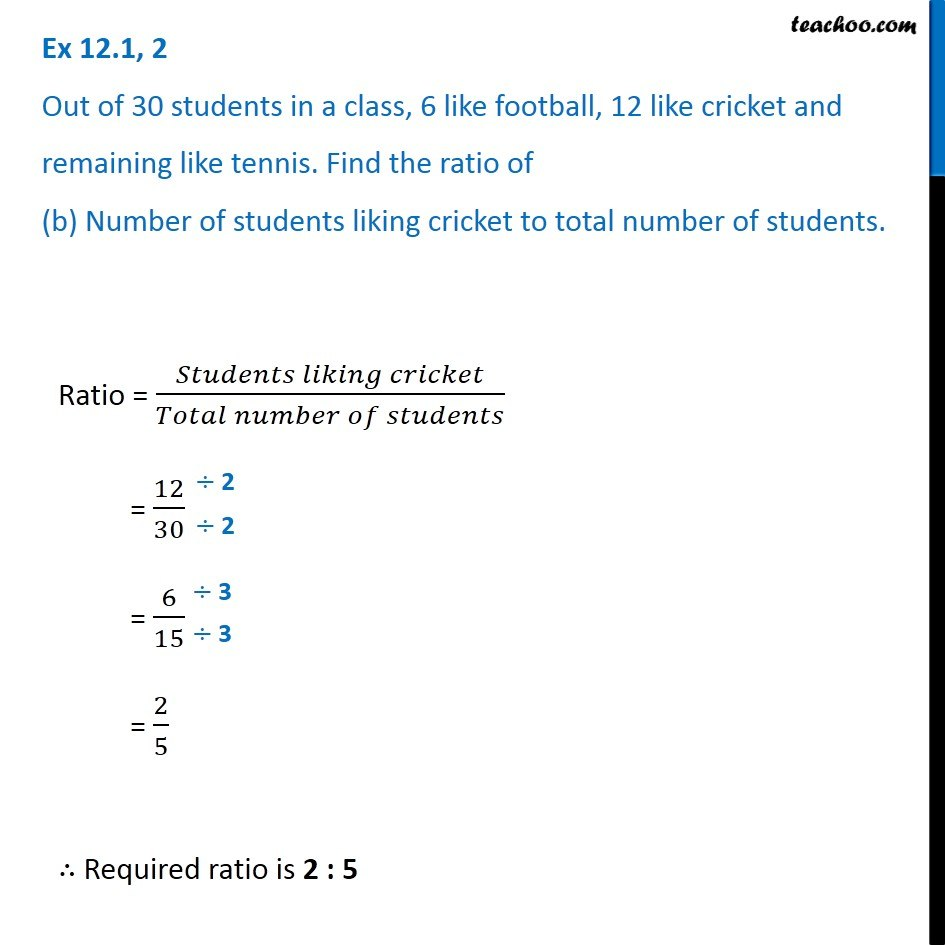Ex 12.1

Chapter 12 Class 6 Ratio and Proportion
Serial order wiseLearn in your speed, with individual attention - Teachoo Maths 1-on-1 Class

### Transcript

Ex 12.1, 2 Out of 30 students in a class, 6 like football, 12 like cricket and remaining like tennis. Find the ratio of (a) Number of students liking football to number of students liking tennis. Total number of students = 30 Students liking football = 6 Students liking cricket = 12 Total number of students = Students like football + Students liking cricket + Students liking tennis 30 = 6 + 12 + Students liking tennis 30 = 18 + Students liking tennis Students liking tennis = 30 − 18 Students liking tennis = 12 Ratio = (𝑆𝑡𝑢𝑑𝑒𝑛𝑡𝑠 𝑙𝑖𝑘𝑖𝑛𝑔 𝑓𝑜𝑜𝑡𝑏𝑎𝑙𝑙)/(𝑆𝑡𝑢𝑑𝑒𝑛𝑡𝑠 𝑙𝑖𝑘𝑖𝑛𝑔 𝑡𝑒𝑛𝑛𝑖𝑠) = 6/12 = 1/2 ∴ Required ratio is 1 : 2 Ex 12.1, 2 Out of 30 students in a class, 6 like football, 12 like cricket and remaining like tennis. Find the ratio of (b) Number of students liking cricket to total number of students. Ratio = (𝑆𝑡𝑢𝑑𝑒𝑛𝑡𝑠 𝑙𝑖𝑘𝑖𝑛𝑔 𝑐𝑟𝑖𝑐𝑘𝑒𝑡)/(𝑇𝑜𝑡𝑎𝑙 𝑛𝑢𝑚𝑏𝑒𝑟 𝑜𝑓 𝑠𝑡𝑢𝑑𝑒𝑛𝑡𝑠) = 12/30 = 6/15 = 2/5 ∴ Required ratio is 2 : 5